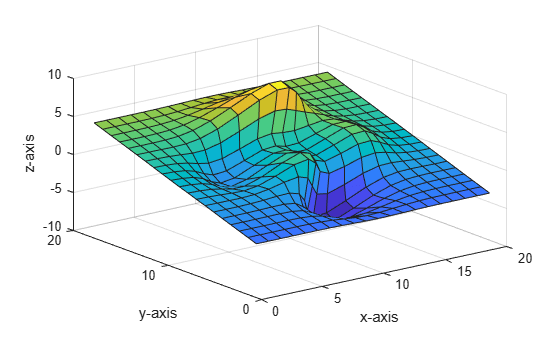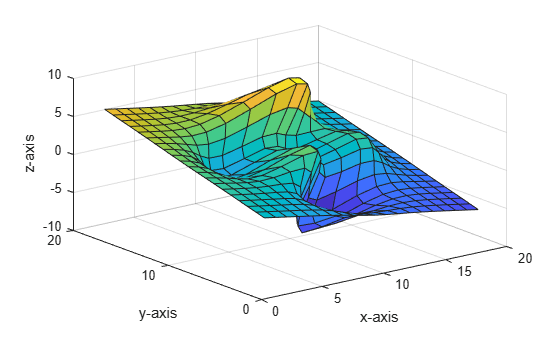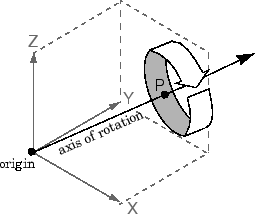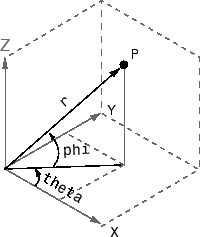# rotate

Rotate object about specified origin and direction

## Syntax

```rotate(h,direction,alpha) rotate(...,origin) ```

## Description

The `rotate` function rotates a graphics object in three-dimensional space.

`rotate(h,direction,alpha)` rotates the graphics object `h` by `alpha` degrees. Specify `h` as a surface, patch, line, text, or image object. `direction` is a two- or three-element vector that describes the axis of rotation in conjunction with the origin of the axis of rotation. The default origin of the axis of rotation is the center of the plot box. This point is not necessarily the origin of the axes.

Positive `alpha` is defined as the righthand-rule angle about the direction vector as it extends from the origin of rotation.

If `h` is an array of handles, all objects must be children of the same axes.

`rotate(...,origin)` specifies the origin of the axis of rotation as a three-element vector` [x0,y0,z0]`.

## Examples

collapse all

Create a surface plot of the `peaks` function and return the surface object.

```s = surf(peaks(20)); xlabel('x-axis') ylabel('y-axis') zlabel('z-axis')```Rotate the surface plot 25 degrees around its x-axis.

```direction = [1 0 0]; rotate(s,direction,25)```Create a surface plot of the `peaks` function and return the surface object.

```s = surf(peaks(20)); xlabel('x-axis') ylabel('y-axis') zlabel('z-axis')```Rotate the surface plot 25 degrees around its y-axis.

```direction = [0 1 0]; rotate(s,direction,25)```Create a surface plot of the `peaks` function and return the surface object.

```s = surf(peaks(20)); xlabel('x-axis') ylabel('y-axis') zlabel('z-axis')```Rotate the surface plot 25 degrees around its x-axis and y-axis.

```direction = [1 1 0]; rotate(s,direction,25)```## Tips

The rotation transformation modifies the object's data. This technique is different from that used by `view` and `rotate3d`, which modify only the viewpoint.

The axis of rotation is defined by an origin of rotation and a point `P`. Specify `P` as the spherical coordinates `[theta phi]` or as the Cartesian coordinates ` [xp,yp,zp]`.In the two-element form for `direction`, `theta` is the angle in the x-y plane counterclockwise from the positive x-axis. `phi` is the elevation of the direction vector from the x-y plane.The three-element form for `direction` specifies the axis direction using Cartesian coordinates. The direction vector is the vector from the origin of rotation to `P`.

## Tips

`rotate` changes the values of the `Xdata`, `Ydata`, and `Zdata` properties to rotate graphics objects.

## Version History

Introduced before R2006a